xHydrostatic equilibriumEncyclopedia
Hydrostatic equilibrium or hydrostatic balance is the condition in fluid mechanics
Fluid mechanics
Fluid mechanics is the study of fluids and the forces on them. Fluid mechanics can be divided into fluid statics, the study of fluids at rest; fluid kinematics, the study of fluids in motion; and fluid dynamics, the study of the effect of forces on fluid motion...

where a volume of a fluid
Fluid
In physics, a fluid is a substance that continually deforms under an applied shear stress. Fluids are a subset of the phases of matter and include liquids, gases, plasmas and, to some extent, plastic solids....

is at rest or at constant velocity. This occurs when compression due to gravity is balanced by a pressure gradient force
The pressure gradient force is not actually a 'force' but the acceleration of air due to pressure difference . It is usually responsible for accelerating a parcel of air from a high atmospheric pressure region to a low pressure region, resulting in wind...

. For instance, the pressure gradient force prevents gravity from collapsing the Earth's atmosphere
Earth's atmosphere
The atmosphere of Earth is a layer of gases surrounding the planet Earth that is retained by Earth's gravity. The atmosphere protects life on Earth by absorbing ultraviolet solar radiation, warming the surface through heat retention , and reducing temperature extremes between day and night...

into a thin, dense shell, while gravity prevents the pressure gradient force from diffusing the atmosphere into space.

Hydrostatic equilibrium is the current distinguishing criterion between dwarf planet
Dwarf planet
A dwarf planet, as defined by the International Astronomical Union , is a celestial body orbiting the Sun that is massive enough to be spherical as a result of its own gravity but has not cleared its neighboring region of planetesimals and is not a satellite...

s and small Solar System bodies
Small Solar System body
A small Solar System body is an object in the Solar System that is neither a planet nor a dwarf planet, nor a satellite of a planet or dwarf planet:...

, and has other roles in astrophysics
Astrophysics
Astrophysics is the branch of astronomy that deals with the physics of the universe, including the physical properties of celestial objects, as well as their interactions and behavior...

and planetary geology
Planetary geology
Planetary geology, alternatively known as astrogeology or exogeology, is a planetary science discipline concerned with the geology of the celestial bodies such as the planets and their moons, asteroids, comets, and meteorites...

. This qualification typically means that the object is symmetrically rounded into a spheroid
Spheroid
A spheroid, or ellipsoid of revolution is a quadric surface obtained by rotating an ellipse about one of its principal axes; in other words, an ellipsoid with two equal semi-diameters....

or ellipsoid shape, where irregular surface features due to a solid, relatively thin, crust
Crust (geology)
In geology, the crust is the outermost solid shell of a rocky planet or natural satellite, which is chemically distinct from the underlying mantle...

are not counted. There are 30 such objects (including the Sun) that are currently confirmed to exist in the Solar System
Solar System
The Solar System consists of the Sun and the astronomical objects gravitationally bound in orbit around it, all of which formed from the collapse of a giant molecular cloud approximately 4.6 billion years ago. The vast majority of the system's mass is in the Sun...

, 7 more which are virtually certain, and a hundred or so more which are likely.

## Mathematical considerationNewton's laws of motion
Newton's laws of motion
Newton's laws of motion are three physical laws that form the basis for classical mechanics. They describe the relationship between the forces acting on a body and its motion due to those forces...

state that a volume of a fluid which is not in motion or which is in a state of constant velocity must have zero net force on it. This means the sum of the forces in a given direction must be opposed by an equal sum of forces in the opposite direction. This force balance is called the hydrostatic balance.

We can split the fluid into a large number of cuboid
Cuboid
In geometry, a cuboid is a solid figure bounded by six faces, forming a convex polyhedron. There are two competing definitions of a cuboid in mathematical literature...

volume elements. By considering just one element, we can work out what happens to the fluid as a whole.

There are 3 forces: the force downwards onto the top of the cuboid from the pressure, P, of the fluid above it is, from the definition of pressure
Pressure
Pressure is the force per unit area applied in a direction perpendicular to the surface of an object. Gauge pressure is the pressure relative to the local atmospheric or ambient pressure.- Definition :...

,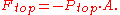Similarly, the force on the volume element from the pressure of the fluid below pushing upwards is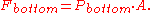Finally, the weight
Weight
In science and engineering, the weight of an object is the force on the object due to gravity. Its magnitude , often denoted by an italic letter W, is the product of the mass m of the object and the magnitude of the local gravitational acceleration g; thus:...

of the volume element causes a force downwards. If the density
Density
The mass density or density of a material is defined as its mass per unit volume. The symbol most often used for density is ρ . In some cases , density is also defined as its weight per unit volume; although, this quantity is more properly called specific weight...

is ρ, the volume is V and g the standard gravity
Standard gravity
Standard gravity, or standard acceleration due to free fall, usually denoted by g0 or gn, is the nominal acceleration of an object in a vacuum near the surface of the Earth. It is defined as precisely , or about...

, then: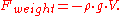The volume of this cuboid is equal to the area of the top or bottom, times the height — the formula for finding the volume of a cube.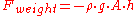By balancing these forces, the total force on the fluid is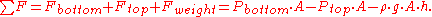This sum equals zero if the fluid's velocity is constant. Dividing by A,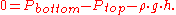Or,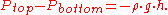Ptop − Pbottom is a change in pressure, and h is the height of the volume element – a change in the distance above the ground. By saying these changes are infinitesimal
Infinitesimal
Infinitesimals have been used to express the idea of objects so small that there is no way to see them or to measure them. The word infinitesimal comes from a 17th century Modern Latin coinage infinitesimus, which originally referred to the "infinite-th" item in a series.In common speech, an...

ly small, the equation can be written in differential
Differential equation
A differential equation is a mathematical equation for an unknown function of one or several variables that relates the values of the function itself and its derivatives of various orders...

form.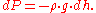Density changes with pressure, and gravity changes with height, so the equation would be: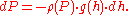Note finally that this last equation can be derived by solving the three-dimensional Navier-Stokes equations
Navier-Stokes equations
In physics, the Navier–Stokes equations, named after Claude-Louis Navier and George Gabriel Stokes, describe the motion of fluid substances. These equations arise from applying Newton's second law to fluid motion, together with the assumption that the fluid stress is the sum of a diffusing viscous...

for the equilibrium situation where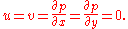Then the only non-trivial equation is the-equation, which now reads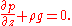Thus, hydrostatic balance can be regarded as a particularly simple equilibrium solution of the Navier-Stokes equations.

### Fluids

The hydrostatic equilibrium pertains to hydrostatics and the principles of equilibrium of fluid
Fluid
In physics, a fluid is a substance that continually deforms under an applied shear stress. Fluids are a subset of the phases of matter and include liquids, gases, plasmas and, to some extent, plastic solids....

s. A hydrostatic balance is a particular balance for weighing substances in water. Hydrostatic balance allows the discovery
Discovery (observation)
Discovery is the act of detecting something new, or something "old" that had been unknown. With reference to science and academic disciplines, discovery is the observation of new phenomena, new actions, or new events and providing new reasoning to explain the knowledge gathered through such...

of their specific gravities
Specific gravity
Specific gravity is the ratio of the density of a substance to the density of a reference substance. Apparent specific gravity is the ratio of the weight of a volume of the substance to the weight of an equal volume of the reference substance. The reference substance is nearly always water for...

.

### Astrophysics

In any given layer of a star
Star
A star is a massive, luminous sphere of plasma held together by gravity. At the end of its lifetime, a star can also contain a proportion of degenerate matter. The nearest star to Earth is the Sun, which is the source of most of the energy on Earth...

, there is a hydrostatic equilibrium between the outward thermal pressure from below and the weight of the material above pressing inward. The isotropic gravitational field compresses the star into the most compact shape possible. A rotating star in hydrostatic equilibrium is an oblate spheroid up to a certain angular velocity. An extreme example of this is the star Vega
Vega
Vega is the brightest star in the constellation Lyra, the fifth brightest star in the night sky and the second brightest star in the northern celestial hemisphere, after Arcturus...

, which has a rotation period of 12.5 hours and is about 20% fatter at the equator than at the poles because of it. Above that critical velocity, it becomes a Jacobi (scalene) ellipsoid, and at still faster rotation it is no longer ellipsoidal at all but piriform
Piriform
Piriform is a privately owned software house based in the West End of London, UK. It develops software for the Microsoft Windows and Mac OS X operating systems. Piriform's projects include CCleaner, Recuva, Defraggler, and Speccy, with at least two more products under development for a future...

, with yet other shapes beyond that.

If the star has a massive nearby companion object then tidal force
Tidal force
The tidal force is a secondary effect of the force of gravity and is responsible for the tides. It arises because the gravitational force per unit mass exerted on one body by a second body is not constant across its diameter, the side nearest to the second being more attracted by it than the side...

s come into play as well, distorting the star into a scalene shape when rotation alone would make it a spheroid. An example of this is Beta Lyrae
Beta Lyrae
Beta Lyrae is a binary star system approximately 882 light-years away in the constellation Lyra. Beta Lyrae has the traditional name Sheliak , from الشلياق šiliyāq, the Arabic name of the constellation Lyra.Beta Lyrae is an eclipsing semi-detached binary system made up of a B7II primary star and...

.

Hydrostatic equilibrium is also important for the intracluster medium
Intracluster medium
In astronomy, the intracluster medium is the superheated plasma present at the center of a galaxy cluster. This is gas heated to temperatures of between roughly 10 and 100 megakelvins and consisting mainly of ionised hydrogen and helium, containing most of the baryonic material in the cluster...

, where it restricts the amount of fluid that can be present in the core of a cluster of galaxies.

### Planetary geology

The concept of hydrostatic equilibrium has also become important in determining whether an astronomical object is a planet
Planet
A planet is a celestial body orbiting a star or stellar remnant that is massive enough to be rounded by its own gravity, is not massive enough to cause thermonuclear fusion, and has cleared its neighbouring region of planetesimals.The term planet is ancient, with ties to history, science,...

, dwarf planet
Dwarf planet
A dwarf planet, as defined by the International Astronomical Union , is a celestial body orbiting the Sun that is massive enough to be spherical as a result of its own gravity but has not cleared its neighboring region of planetesimals and is not a satellite...

, or small Solar System body
Small Solar System body
A small Solar System body is an object in the Solar System that is neither a planet nor a dwarf planet, nor a satellite of a planet or dwarf planet:...

. According to the definition of planet
Definition of planet
The definition of planet, since the word was coined by the ancient Greeks, has included within its scope a wide range of celestial bodies. Greek astronomers employed the term asteres planetai , "wandering stars", for objects which apparently move over the sky...

adopted by the International Astronomical Union
International Astronomical Union
The International Astronomical Union IAU is a collection of professional astronomers, at the Ph.D. level and beyond, active in professional research and education in astronomy...

in 2006, planets and dwarf planets are objects that have sufficient gravity to overcome their own rigidity and assume hydrostatic equilibrium. Sometimes this means a spheroid. However, in the cases of moons in synchronous orbit, near unidirectional tidal forces create a scalene ellipsoid, and the dwarf planet also appears to be scalene due to its rapid rotation.

In the Solar System, it appears that icy objects with a diameter larger than ca 400 km are usually in hydrostatic equilibrium, while those smaller than that are not. Icy objects, though, can achieve hydrostatic equilibrium at a smaller size than rocky objects. The smallest object in hydrostatic equilibrium is the icy moon Mimas
Mimas (moon)
Mimas is a moon of Saturn which was discovered in 1789 by William Herschel. It is named after Mimas, a son of Gaia in Greek mythology, and is also designated Saturn I....

at 397 km, while the largest object not in hydrostatic equilibrium is the rocky asteroid Pallas
2 Pallas
Pallas, formally designated 2 Pallas, is the second asteroid to have been discovered , and one of the largest. It is estimated to constitute 7% of the mass of the asteroid belt, and its diameter of 530–565 km is comparable to, or slightly larger than, that of 4 Vesta. It is however 20%...

at 532 km.

Since the terrestrial planet
Terrestrial planet
A terrestrial planet, telluric planet or rocky planet is a planet that is composed primarily of silicate rocks or metals. Within the Solar System, the terrestrial planets are the inner planets closest to the Sun...

s and dwarf planets (and likewise the larger satellites
Natural satellite
A natural satellite or moon is a celestial body that orbits a planet or smaller body, which is called its primary. The two terms are used synonymously for non-artificial satellites of planets, of dwarf planets, and of minor planets....

, like the Moon
Moon
The Moon is Earth's only known natural satellite,There are a number of near-Earth asteroids including 3753 Cruithne that are co-orbital with Earth: their orbits bring them close to Earth for periods of time but then alter in the long term . These are quasi-satellites and not true moons. For more...

and Io
Io (moon)
Io ) is the innermost of the four Galilean moons of the planet Jupiter and, with a diameter of , the fourth-largest moon in the Solar System. It was named after the mythological character of Io, a priestess of Hera who became one of the lovers of Zeus....

) have irregular surfaces, this definition evidently has some flexibility, but as of yet a specific means of quantifying an object's shape by this standard has not been announced. Local irregularities may be consistent with global equilibrium. For example, the massive base of the tallest mountain on Earth, Mauna Kea
Mauna Kea
Mauna Kea is a volcano on the island of Hawaii. Standing above sea level, its peak is the highest point in the state of Hawaii. However, much of the mountain is under water; when measured from its oceanic base, Mauna Kea is over tall—significantly taller than Mount Everest...

, has deformed and depressed the level of the surrounding crust, so that the overall distribution of mass approaches equilibrium. The amount of leeway afforded the definition could affect the classification of the asteroid Vesta
4 Vesta
Vesta, formally designated 4 Vesta, is one of the largest asteroids, with a mean diameter of about . It was discovered by Heinrich Wilhelm Olbers on March 29, 1807, and is named after the Roman virgin goddess of home and hearth, Vesta....

, which may have solidified while in hydrostatic equilibrium but was subsequently significantly deformed by large impacts.

### Atmospherics

In the atmosphere, the pressure of the air decreases with increasing altitude. This pressure difference causes an upward force called the pressure gradient force
The pressure gradient force is not actually a 'force' but the acceleration of air due to pressure difference . It is usually responsible for accelerating a parcel of air from a high atmospheric pressure region to a low pressure region, resulting in wind...

. The force of gravity balances this out, keeping the atmosphere bound to the earth and maintaining pressure differences with altitude.